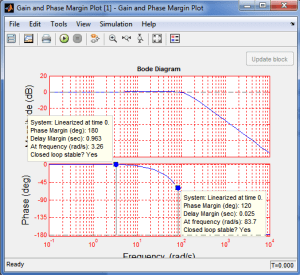# How to Setup Bode Plot in Simulink

Below is the step by step procedure on how to setup bode plot in Simulink. Bode plot is a graph of gain and phase of a system. In a bode plot you can get the gain and phase margins and know if the system is stable or not.

To demonstrate this properly, consider the Simulink model in Figure 1. You should have license for Simulink control design to use the bode plot. Also in this tutorials I used Matlab R2012b. Higher versions of Matlab may have slight different interface and you need a little exploration.

## 1. Get the Gain and Phase Margin Plot

• In the Simulink Library Browser, navigate to Simulink Control Design and get “Gain and Phase Margin Plot” under “Linear Analysis Ports”.
• Drag the Gain and Phase Margin Plot to the Simulink model in Figure 1.

## 2. Setup Linear Analysis Points

• Right click on the line between the transfer function and the scope. Navigate to “Linear Analysis Points” then click “Output Point”. This simply means that we are declaring this section as the output.
• Right click on the line between the “Step” input and the “Summer” then navigate to “Linear Analysis Points” then click “Input Point”
• After putting input and output points, this is the result

As you can see there is a small arrow beside Step input and transfer function.

## 3. Setting up the Gain and Phase Margin Plot

• Double click on the “Gain and Phase Margin Plot” and this should be the window content
• Tick on the “Show plot on block open” and then click “Apply” then “OK”.
• After clicking “OK”, the window will disappear. Double click again the “Gain and Phase Margin Plot” and you can see this

• Click on the item in red box below
• The magnitude and phase plot should show up as below.

## 4. Viewing the Gain and Phase Margins

• To view the gain and phase margins, right click on the plot then hover to “Characteristics” then “All Stability Margins”.